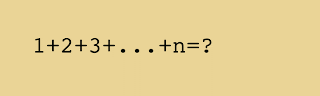## Thursday, July 11, 2019

### Zero probability

In a previous post I mentioned that an event can happen once or several times, although the probability of its happening is zero. The probability of an event is defined as the ratio of the number of favorable cases to that of possible cases. Therefore, if the number of possible cases is infinite, while that of favorable cases is finite, the probability turns out to be zero.
At first glance it seems incredible that an event with zero probability can actually happen. I think the matter will be clearer with a simple example. Two friends, A and B, are talking, and what they say is this:
A: If I ask you to choose a number between 1 and 100, what is the probability that you choose a specific number, such as 25?
B: 1/100, obviously.
A: If I ask you to choose a number between 1 and 1000, what is the probability that you choose 25?
B: 1/1000.
A: If I ask you to choose a number between 1 and 10,000, what is the probability that you choose 25?
B: 1/10,000.
A: If I ask you to choose a positive integer number, what is the probability that you choose 25?
B: Zero, for the set of integers has infinite elements, and one divided by infinity is equal to zero.
A: Choose any number among all the positive integers and tell me which number you have chosen.
B: I choose 22500-1.
A: You have just made an event with zero  probability.
Thinking a little you’ll see that the probability of choosing, among all the integers, any finite set, however large, is also zero. For instance:
A: If I ask you to choose ten different numbers between one and one hundred, what is the probability that you choose precisely the numbers between 11 and 20? (their order does not matter)
B: 1 / 17,310,309,456,440
A: And if I ask you to choose ten different numbers among all the positive integers, what is the probability that you choose precisely the numbers between 11 and 20?
B: Zero.
I leave to the curious reader to compute why the probability of choosing numbers 11 to 20 among those from one to one hundred is precisely what B has stated.
To finish this post, I’ll propose a few more exercises for the reader. Whoever solves them has the opportunity to write a comment explaining how they arrived to the solution.
1.      What is the last digit of 62500?
2.      What is the penultimate digit of 62500?
3.      What is the penultimate digit of 61,000,000?
4.      What is the probability that the last digit of 6n is odd?
5.      What is the probability that the penultimate digit of 6n is odd?By Vincent Pantaloni, CC BY-SA 4.0, Wikimedia Commons

The same post in Spanish
Thematic Thread on Statistics: Previous Next
Manuel Alfonseca
Happy summer holidays. See you by mid-August